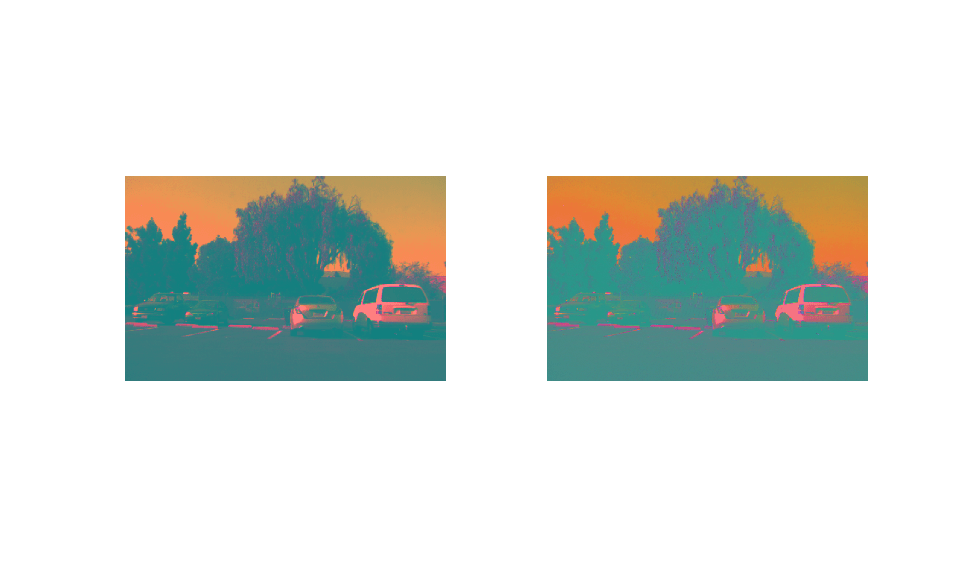# SystemC Code Generation for Image Format Conversion from RGB to YUV

This example shows how to generate SystemC™ code from a MATLAB® design that converts the image format from RGB to YUV.

### MATLAB Design and Test Bench

Set up the `Rgb2yuv` model for this example.

```mlhdlc_demo_setup('Rgb2yuv'); design_name = 'mlhdlc_rgb2yuv'; testbench_name = 'mlhdlc_rgb2yuv_tb'; ```
```no files copied to tempdir... ```

Review the `Rgb2yuv` design.

```open(design_name) ```
```function [x_out, y_out, y_data_out, u_data_out, v_data_out] = ... mlhdlc_rgb2yuv(x_in, y_in, r_in, g_in, b_in) %#codegen % Copyright 2011-2019 The MathWorks, Inc. persistent RGB_Reg YUV_Reg persistent x1 x2 y1 y2 if isempty(RGB_Reg) RGB_Reg = zeros(3,1); YUV_Reg = zeros(3,1); x1 = 0; x2 = 0; y1 = 0; y2 = 0; end D = [.299 .587 .144; -.147 -.289 .436; .615 -.515 -.1]; C = [0; 128; 128]; RGB = [r_in; g_in; b_in]; YUV_1 = D*RGB_Reg; YUV_2 = YUV_1 + C; RGB_Reg = RGB; y_data_out = round(YUV_Reg(1)); u_data_out = round(YUV_Reg(2)); v_data_out = round(YUV_Reg(3)); YUV_Reg = YUV_2; x_out = x2; x2 = x1; x1 = x_in; y_out = y2; y2 = y1; y1 = y_in; ```

Review the `Rgb2yuv` test bench:

```open(testbench_name); ```
```FRAMES = 1; WIDTH = 752; HEIGHT = 480; HBLANK = 10;%748; VBLANK = 10;%120; % Copyright 2011-2019 The MathWorks, Inc. vidData = double(imread('mlhdlc_img_yuv.tif')); for f = 1:FRAMES vidOut = zeros(HEIGHT, WIDTH, 3); for y = 0:HEIGHT+VBLANK-1 for x = 0:WIDTH+HBLANK-1 if y >= 0 && y < HEIGHT && x >= 0 && x < WIDTH b = vidData(y+1,x+1,1); g = vidData(y+1,x+1,2); r = vidData(y+1,x+1,3); else b = 0; g = 0; r = 0; end [xOut, yOut, yData, uData, vData] = ... mlhdlc_rgb2yuv(x, y, r, g, b); if yOut >= 0 && yOut < HEIGHT && xOut >= 0 && xOut < WIDTH vidOut(yOut+1,xOut+1,:) = [yData vData uData]; end end end figure(1); subplot(1,2,1); imshow(uint8(vidData)); subplot(1,2,2); imshow(ycbcr2rgb(uint8(vidOut))); drawnow; end ```

### Test the MATLAB Algorithm

To avoid run-time errors, simulate the design with the test bench.

```mlhdlc_rgb2yuv_tb ```### Create HDL Coder™ Project

To generate SystemC code from a MATLAB design:

1. Create a HDL Coder project:

```coder -hdlcoder -new mlhdlc_rgb_prj ```

2. Add the file `mlhdlc_rgb2yuv.m` to the project as the MATLAB Function and `mlhdlc_rgb2yuv_tb.m` as the MATLAB Test Bench.

3. Click Autodefine types to use the recommended types for the inputs and outputs of the MATLAB function `mlhdlc_rgb2yuv`.

### Run Fixed-Point Conversion and SystemC Code Generation

To generate SystemC code from the MATLAB design:

1. At the MATLAB command line, set up the path for SystemC code generation by using the function `hdlsetuphlstoolpath`.